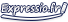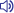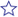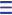multiply translation | English-French dictionary

Search also in: Web News Encyclopedia Images Contextmultiply

vt
[+number]
multiplier
to multiply 6 by 3      multiplier six par trois
to multiply sth by sth      multiplier qch par qch
→ Multiply six by three.
→ two multiplied by three is six.
→ ... if you multiply seven and six
→ their remarkable ability to multiply huge numbers
vi
(=proliferate)   se multiplier
→ Such disputes multiplied in the eighteenth and nineteenth centuries.
→ The creatures began to multiply very rapidly.
(=do multiplication sums)   faire des multiplications
→ We encourage students to multiply and divide without using a calculator
multiplyv.multiplier (vt.) ; se multiplier (vr.)multiply upv. multiplieradj. multirésistant [Med.]n. unité de multiplication et de division [Comp.]exp. multiplier par 3exp. multiplier six par troisexp. multiplier qch par qch'multiply' also found in translations in French-English dictionary multiplierv. multiplyse démultiplierv. multiply[Med.]décuplerv. multiply tenfoldn. multiply handicapped personn. multiply handicapped personsextuplerv. multiply by six (vt.) ; increase sixfold (vi.)